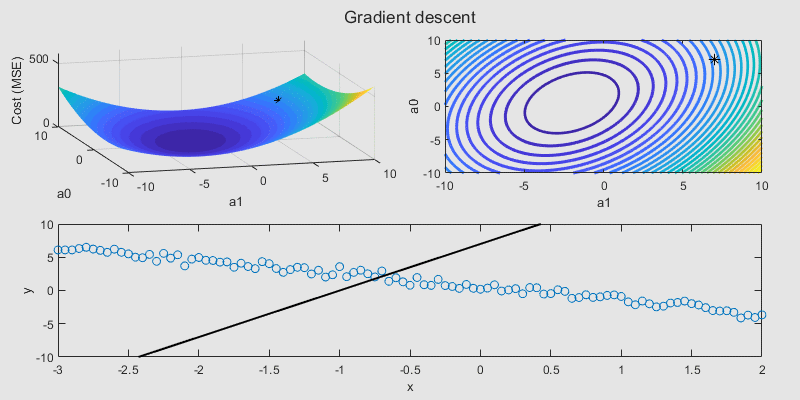Regression Basics

version 2.0.0 (285 KB) by
Interactive courseware module that addresses the fundamentals of regression analysis taught in STEM courses.

Updated 06 Aug 2021

From GitHub

Regression BasicsCurriculum Module
Created with R2020a. Compatible with R2020a and later releases.

Description

This package contains a live script and supporting files to illustrate some basics of regression analysis. The materials are designed to be flexible and can be easily modified to accommodate a variety of teaching and learning methods. We include a brief background, interactive illustrations, tasks, reflection questions, a real-world application example, and a guided exercise for the different concepts explored.

The instructions inside the live scripts will guide you through the tasks and activities one section at a time. To run this interactive script in a controls-only mode, use the Hide code button on the View tab of the MATLAB toolstrip.

Learning Goals

• Explain the difference between linear, multiple linear, and nonlinear regression.
• Use ordinary least squares to solve for linear regression parameters.
• Assess and improve the performance of a regression model using a goodness-of-fit measure.
• Apply gradient descent to iteratively minimize a cost function and estimate model parameters.
• Explain the effect of increasing and decreasing the learning rate and number of steps for the gradient descent algorithm.
• Use a linear regression model to perform short-term forecasting.Suggested Prework

MATLAB Onramp – a free two-hour introductory tutorial to learn the essentials of MATLAB®.

This module assumes a basic knowledge of algebra and multivariable calculus.

Details

regressionBasics.mlx
An interactive lesson that introduces the fundamentals of regression analysis. Students apply a basic linear regression to model real-world electricity load data.

A supplementary script to download the external electricity load data from New York ISO for use in the practice problem.

regressSolnIm/
This folder includes supplementary figure files containing solutions for tasks in regressionBasics.mlx. The main script provides controls to hide or expose the solutions when needed. Ensure that this folder is in the same location as regressionBasics.mlx

linearData.mat, linearData2.mat, multivariateData.mat, nonlinearData.mat
Data files containing some sample data for the different types of regression problems.

Products

MATLAB, Statistics and Machine Learning Toolbox™

The license for this module is available in the LICENSE.TXT file in this GitHub repository.

Educator Resources

Have any questions or feedback? Contact the MathWorks online teaching team.

Cite As

Emma Smith Zbarsky (2022). Regression Basics (https://github.com/MathWorks-Teaching-Resources/Regression-Basics/releases/tag/v2.0.0), GitHub. Retrieved .

MATLAB Release Compatibility
Created with R2020a
Compatible with R2020a and later releases
Platform Compatibility
Windows macOS Linux